## 不用strlen 该怎么样算出一个PHP字符串的长度 求解答

walden 发布于 2012/03/05 21:33

1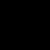#### 引用来自“simonXia”的答案

<?php

\$str = "adfa我是0 大大大adf \

";

echo strlen(\$str),"\n";

while (isset(\$str{++\$i})) {

}

echo \$i;
?>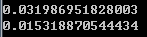<?php

\$str = "adfa我是0 大大大adf \

";
\$start1=microtime(true);
for(\$a=0;\$a<100000;\$a++){
strlen(\$str);
}
echo microtime(true)-\$start1,"\n";
\$start2=microtime(true);
for(\$a=0;\$a<100000;\$a++){
while (isset(\$str{++\$i})) {

}
}
echo microtime(true)-\$start2,"\n";
?>

`while (isset(\$str{++\$i}));`

`while ( isset(\$str{\$i}) && ++\$i );`

php生成字符窜的时候会把长度保存到str结构体的len字段上, 而php的strlen并不是遍历,只是取这个字段, 所以效率上绝对远远高过其他方式

00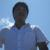<?php

\$str = "adfa我是0 大大大adf \

";

echo strlen(\$str),"\n";

while (isset(\$str{++\$i})) {

}

echo \$i;
?><?php

\$str = "adfa我是0 大大大adf \

";
\$start1=microtime(true);
for(\$a=0;\$a<100000;\$a++){
strlen(\$str);
}
echo microtime(true)-\$start1,"\n";
\$start2=microtime(true);
for(\$a=0;\$a<100000;\$a++){
while (isset(\$str{++\$i})) {

}
}
echo microtime(true)-\$start2,"\n";
?>0#### 引用来自“simonXia”的答案

<?php

\$str = "adfa我是0 大大大adf \

";

echo strlen(\$str),"\n";

while (isset(\$str{++\$i})) {

}

echo \$i;
?><?php

\$str = "adfa我是0 大大大adf \

";
\$start1=microtime(true);
for(\$a=0;\$a<100000;\$a++){
strlen(\$str);
}
echo microtime(true)-\$start1,"\n";
\$start2=microtime(true);
for(\$a=0;\$a<100000;\$a++){
while (isset(\$str{++\$i})) {

}
}
echo microtime(true)-\$start2,"\n";
?>

`while (isset(\$str{++\$i}));`

`while ( isset(\$str{\$i}) && ++\$i );`

php生成字符窜的时候会把长度保存到str结构体的len字段上, 而php的strlen并不是遍历,只是取这个字段, 所以效率上绝对远远高过其他方式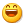0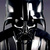#### 引用来自“simonXia”的答案

<?php

\$str = "adfa我是0 大大大adf \

";

echo strlen(\$str),"\n";

while (isset(\$str{++\$i})) {

}

echo \$i;
?><?php

\$str = "adfa我是0 大大大adf \

";
\$start1=microtime(true);
for(\$a=0;\$a<100000;\$a++){
strlen(\$str);
}
echo microtime(true)-\$start1,"\n";
\$start2=microtime(true);
for(\$a=0;\$a<100000;\$a++){
while (isset(\$str{++\$i})) {

}
}
echo microtime(true)-\$start2,"\n";
?>

`while (isset(\$str{++\$i}));`

`while ( isset(\$str{\$i}) && ++\$i );`

php生成字符窜的时候会把长度保存到str结构体的len字段上, 而php的strlen并不是遍历,只是取这个字段, 所以效率上绝对远远高过其他方式